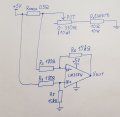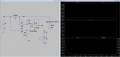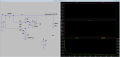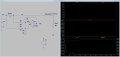# Problem with current sense circuit using opamp

#### Vilius_Zalenas

Joined Jul 24, 2022
109
Hi,

I am a beginner in hardware design. Recently got a task to design a simple current measurement device. The device should have only 3 states:

Current is lower than 10 mA
Current is between 10 and 100 mA
Current is higher than 100 mA

For current measurement I am using a LM358N op amp in a differential amplifier configuration (seems like a very simple circuit.) Since I am only interested in the mentioned range, I calculated the resistor values to provide an amplification factor of around 90. This should result in op amp output voltage of roughly 0.5 - 4.5V respectively at 10 - 100 mA of current flowing in the main branch.

For testing purposes, I connected a high power potentiometer (which should simulate different current consumption) in series with a 10 Ohm 10W limiting resistor ( if the pot was turned all the way down to zero.) According to the theory, increased current should result in a higher voltage drop across the resistor, thus increasing the op amps output voltage.

However, I am getting everything but the desired result. When the potentiometer is virtually turned to short circuit, I am getting around 4.08 V at the op amps output, when I turn potentiometer to the 330 Ohm (max value), my output is at 4.66 V.

First of all, increased resistance should result in decreased current, so the output voltage should also decrease (I am getting opposite result)
Second, the output voltage range is just over 0.5 V, while according to the formula range should be no less than 4 V

Do you have any idea, what could be causing the problem?

Some other details:
I made this circuit on a breadboard (I understand that every component has its own tolerance, so as the op amp does, but there is no AC component in this circuit. I could understand 10-15 % error, but in my case its much worse)
I checked my wiring like a 100 times.
I have a really generous amount of bypass caps.
I have tried two LM358N ICs, so the guess that one could have been defected does not hold...

(Not sure if the whole text was formatted right, please, let me know)Last edited by a moderator:

#### ScottWang

Joined Aug 23, 2012
7,342

#### MrSalts

Joined Apr 2, 2020
2,767
Check that your pot actually goes from 0-330ohms. Full range. If it does not go all the way to 330ohms, you'll have trouble getting low values. Also, make sure the pot is linear. If you're using some old pot from a junk drawer, it may be scorched somewhere and not a smooth increase of resistance through the turn.

finally, the output of the LM358 is only 0-3V when using a 5v supply with a 2k ohm load. You may need some load to ground at the output.

#### Alec_t

Joined Sep 17, 2013
13,443
You are using the LM358 with inputs beyond its common mode range. At maximum they should be about 2V below the supply voltage.

#### BobTPH

Joined Jun 5, 2013
6,527
Even if your circuit worked correctly, how does it solve the original problem? How does it output the three states it is supposed to?

You are going about this completely wrongly. You need a circuit that will discriminate the three states you identified. Think 3 LEDs, with only one of them lighting for any given current.

#### MrSalts

Joined Apr 2, 2020
2,767
Even if your circuit worked correctly, how does it solve the original problem? How does it output the three states it is supposed to?

You are going about this completely wrongly. You need a circuit that will discriminate the three states you identified. Think 3 LEDs, with only one of them lighting for any given current.
It can be done with two LEDs.

#### MrSalts

Joined Apr 2, 2020
2,767
If your gain is 90, and max output with a max output is 3v (with a 2k load), then the max drop across your sense resistor should be 33mV. And max current through the resistor should be 16.5 mA before you see saturation. Assuming no load, the output may go higher but that "higher level" is not specified on the datasheet so who knows. Designing for a 3v max output is a better idea.

#### crutschow

Joined Mar 14, 2008
32,067
As noted by Alec, you are exceeding the input common-mode voltage range of about 2V below the supply or 3V for the LM358.

If you use a rail-rail type op amp such as an LMC6482 or OPA197, then it should work.

Another option is to increase the input resistor values to 10k which will reduce the common-mode voltage to 3V (along with reducing the gain to 1..5) and then use the other LM358 at the output as a non-inverting amp with a gain of about 56.
But you maximum output would still be about 3V.

You really need to learn how to read data sheets.•BobaMosfet

#### Vilius_Zalenas

Joined Jul 24, 2022
109
You are using the LM358 with inputs beyond its common mode range. At maximum they should be about 2V below the supply voltage.
So 180 Ohm and 15K resistor voltage divider results in voltage of almost 5V at my non inverting input. Are you saying that if I supply the op amp with 5V, voltage at the input can not be higher than 3V? In that case my whole design is worth nothing...

#### Alec_t

Joined Sep 17, 2013
13,443
Are you saying that if I supply the op amp with 5V, voltage at the input can not be higher than 3V?
Yes.
Crutschow has indicated how you can overcome this (post #8).

#### Vilius_Zalenas

Joined Jul 24, 2022
109
As noted by Alec, you are exceeding the input common-mode voltage range of about 2V below the supply or 3V for the LM358.

If you use a rail-rail type op amp such as an LMC6482 or OPA197, then it should work.

Another option is to increase the input resistor values to 10k which will reduce the common-mode voltage to 3V (along with reducing the gain to 1..5) and then use the other LM358 at the output as a non-inverting amp with a gain of about 56.
But you maximum output would still be about 3V.

You really need to learn how to read data sheets.Could I do it on a single chip ? LM358N PDIP8 package has 2 op amps with common supply rail, would it work?

#### Alec_t

Joined Sep 17, 2013
13,443
Yes, provided a 3V output is sufficent for your needs.

#### Irving

Joined Jan 30, 2016
3,435
Your problem is that the formula assumes a perfect opamp and infinite voltages. Neither is true.

Consider the voltage at the opamp + input; its 5v * 15k/(15k+180) = 4.94v

Now consider the -ve input. At 1A current the voltage at the RH end of Rsense will be 4.5v so for the opamp to make its two inputs equal the output must be higher than 4.5v , something like 36v!

Even a rail-to-rail opamp won't work properly below about 15mA, you need a -ve rail for the opamp or a small bias on the +ve input.

Here's a simulation with your LM358, a gain of 2 and a dual supply, so keeping it within common-mode parameters. Now 1v out = 1AAnd here with a voltage offset and a single supply:#### Alec_t

Joined Sep 17, 2013
13,443
Another option using a LM358 is to have a low-side current shunt instead of your high-side shunt, since ground is within the common-mode input range.

#### Vilius_Zalenas

Joined Jul 24, 2022
109
As noted by Alec, you are exceeding the input common-mode voltage range of about 2V below the supply or 3V for the LM358.

If you use a rail-rail type op amp such as an LMC6482 or OPA197, then it should work.

Another option is to increase the input resistor values to 10k which will reduce the common-mode voltage to 3V (along with reducing the gain to 1..5) and then use the other LM358 at the output as a non-inverting amp with a gain of about 56.
But you maximum output would still be about 3V.

You really need to learn how to read data sheets.So swapped the input resistor to 10k (instead of 180 Ohm before). However, now I always get 0.7V at the output, no matter the potentiometer value... (Potentiometer is tested and working well)

#### MrAl

Joined Jun 17, 2014
10,091
Hi,

I am a beginner in hardware design. Recently got a task to design a simple current measurement device. The device should have only 3 states:

Current is lower than 10 mA
Current is between 10 and 100 mA
Current is higher than 100 mA

For current measurement I am using a LM358N op amp in a differential amplifier configuration (seems like a very simple circuit.) Since I am only interested in the mentioned range, I calculated the resistor values to provide an amplification factor of around 90. This should result in op amp output voltage of roughly 0.5 - 4.5V respectively at 10 - 100 mA of current flowing in the main branch.

For testing purposes, I connected a high power potentiometer (which should simulate different current consumption) in series with a 10 Ohm 10W limiting resistor ( if the pot was turned all the way down to zero.) According to the theory, increased current should result in a higher voltage drop across the resistor, thus increasing the op amps output voltage.

However, I am getting everything but the desired result. When the potentiometer is virtually turned to short circuit, I am getting around 4.08 V at the op amps output, when I turn potentiometer to the 330 Ohm (max value), my output is at 4.66 V.

First of all, increased resistance should result in decreased current, so the output voltage should also decrease (I am getting opposite result)
Second, the output voltage range is just over 0.5 V, while according to the formula range should be no less than 4 V

Do you have any idea, what could be causing the problem?

Some other details:
I made this circuit on a breadboard (I understand that every component has its own tolerance, so as the op amp does, but there is no AC component in this circuit. I could understand 10-15 % error, but in my case its much worse)
I checked my wiring like a 100 times.
I have a really generous amount of bypass caps.
I have tried two LM358N ICs, so the guess that one could have been defected does not hold...

(Not sure if the whole text was formatted right, please, let me know)View attachment 288429
Hi there,

For the use of the LM358 you could use one of the solutions already given in this thread OR you could go with ground current sensing. That would put the resistor in series with the ground of the load so you eliminate the problem with sensing near the power supply voltage. There are still a few other issues.

As also mentioned in this thread, the output can only go to about Vcc-1.5v which puts it at max 3.5 volts for Vcc=5 volts.
If that is not good enough, there is a trick you can use to get near 5v output but then you cant go all the way to ground (without an output transistor that is).
If you do add an output transistor you can get a wide output voltage range.

Also, the LM358 is a great op amp and has been around ever since i can remember, almost, but it does have an input offset that might be objectionable in this kind of application because you need high gain, nearly 100. That means with an input offset of 2mv you would get 0.200 volts at the output even with zero current unless it happened to be a negative input offset, which is less likely, and then you would not get any output until your current reached the level where it could cause over 0.200 volts on the output. You could bias the input to help get rid of the input offset. There may be a circuit for this on the web or else i can provide one.

A better solution, and probably the best solution, is to use a chopper stabilized amplifier. That gives you very very low input offset so much less error. That's often the best for measuring DC current.

I actually used the LM358 in a current measuring application and i can tell you it does work, but you have to be prepared to deal with the input offset. If you have a positive offset you might get away with just subtracting the output offset from your reading. I did that by inspection of the meter movement i was using so that is a possibility but not very elegant.

So to summarize:
1. Input common mode problem, maybe you can sense the ground current of the load instead which would be very typical, or get a rail to rail input op amp.
2. Input offset, provide an input bias network possibly just one resistor, or go to a chopper stabilized op amp.
3. Output voltage range, add a transistor although you may also have to add a compensation network, or get a rail to rail output op amp.

There are a lot of other possibilities. For example, there are IC chips made specifically for high side current measurements. They are made for this.

I see you did not yet add range selection. You might do that with some comparators and special signal level relays. If you dont need high accuracy maybe MOSFETs or even an analog switch IC chip.

#### Irving

Joined Jan 30, 2016
3,435
So swapped the input resistor to 10k (instead of 180 Ohm before). However, now I always get 0.7V at the output, no matter the potentiometer value... (Potentiometer is tested and working well)
You've still got the common mode problem... here's the simulation with 10k; the opamp goes into a strange state called inversion when the inputs are too close to the supply rail OR the maximum output voltage or you're trying toi force the output higher than its able to go.#### crutschow

Joined Mar 14, 2008
32,067
So swapped the input resistor to 10k (instead of 180 Ohm before). However, now I always get 0.7V at the output, no matter the potentiometer value... (Potentiometer is tested and working well)
Yes, that is another problem with the LM358, it does not pull the output down to 0V with a load (here the feedback resistor).
A higher value of feedback resistor will reduce (but not eliminate that problem)
Using one of the rail-rail op amps I mentioned can pull the output closer to zero.